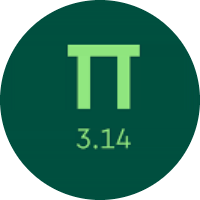# Learning the Statistics by Quiz

In this quiz, you will get to know about the basics of statistics. You will learn about mode, mean, median, range, and mean absolute deviation. You will find different types of problems on these concepts.

Start Quiz

Jon counted the number of fish kept in 7 tanks at the pet store. He counted:What was the range of the numbers of fish?

5

6

4

Sandy prepared and shipped a variety of gift baskets that had been ordered. The 7 packages weighed:What was the mode of the weights?

6

8

5

What is the median?4

5

6

7

Clare has the following data:If the mode is 7, which number could m be?

2

6

7

8

Valentina has the following data:If the median is 4, which number could b be?

6

7

5

4

Lara has the following data:If the range is 6, which number could r be?

10

19

17

Look at this set of 6 numbers:How would the mean change if the number 25 replaced the number 73 in the set?

increase

decrease

no change

Look at this set of 8 numbers:How would the range change if the number 45 replaced the number 10 in the set?

increase

decrease

no change

In the data set below, what is the mean absolute deviation?If the answer is a decimal, round it to the nearest tenth.

0.9

0.6

0.4

In the data set below, what is the lower quartile?1

2

4

Quiz/Test Summary
Title: Learning the Statistics by Quiz
Questions: 10
Contributed by: